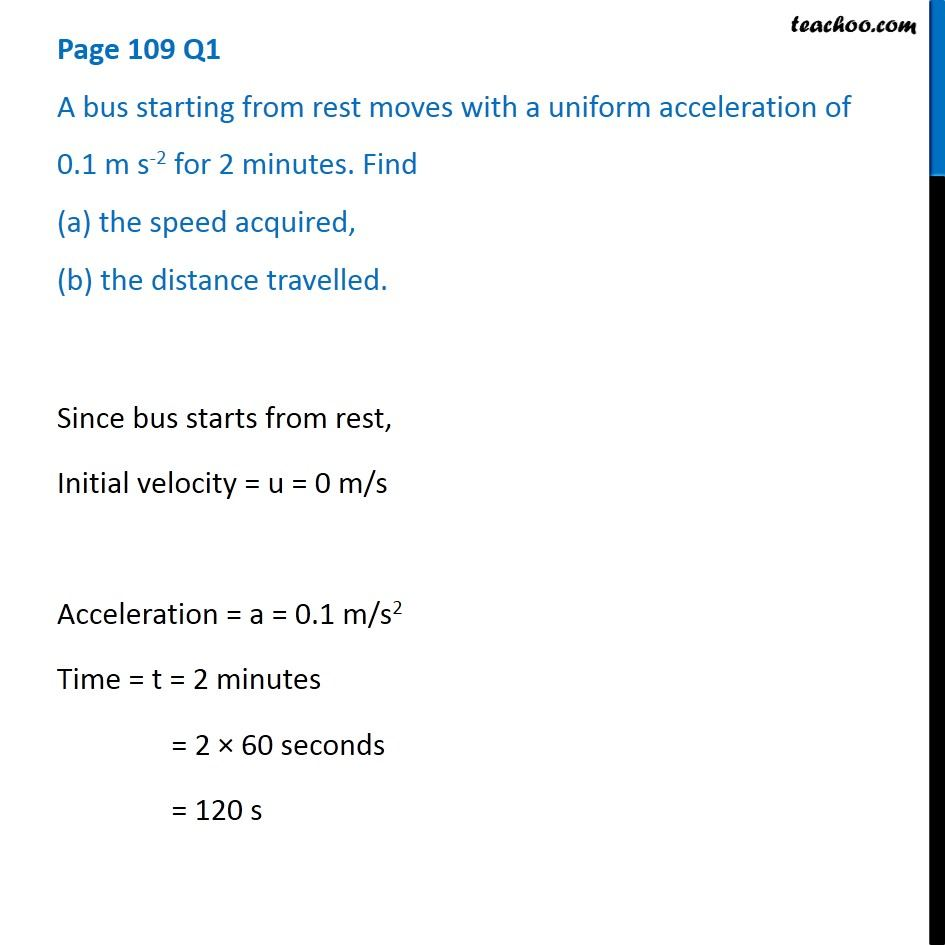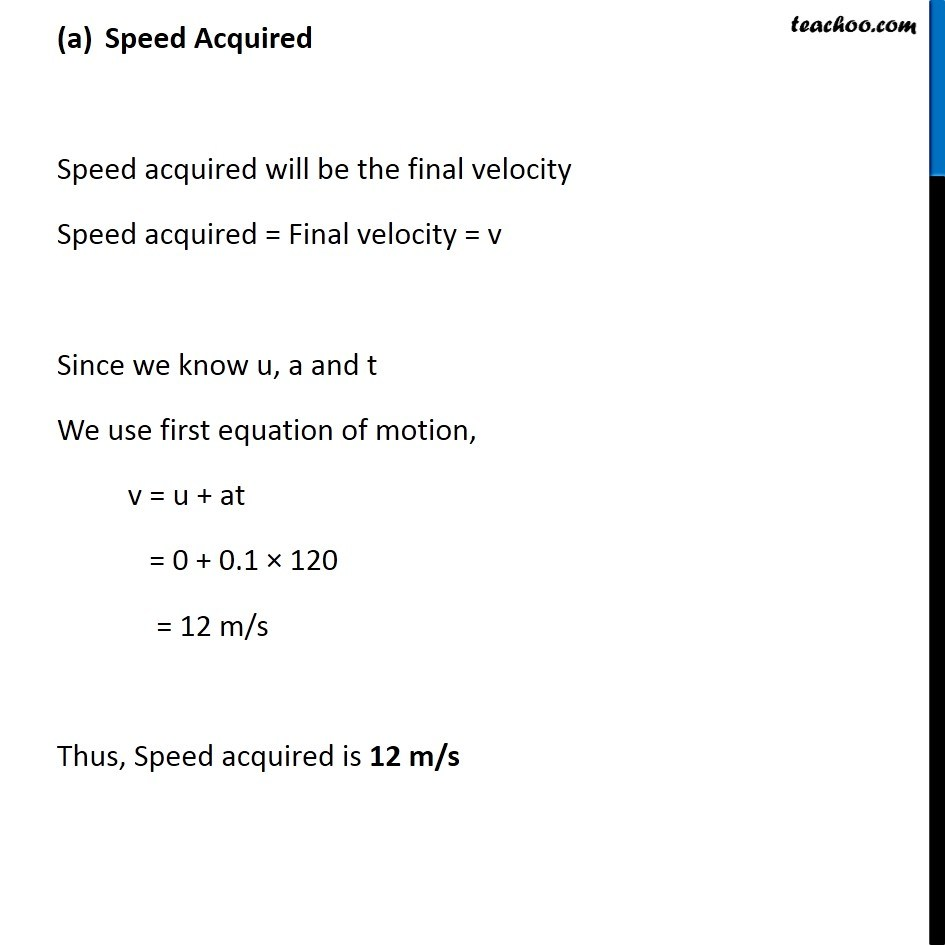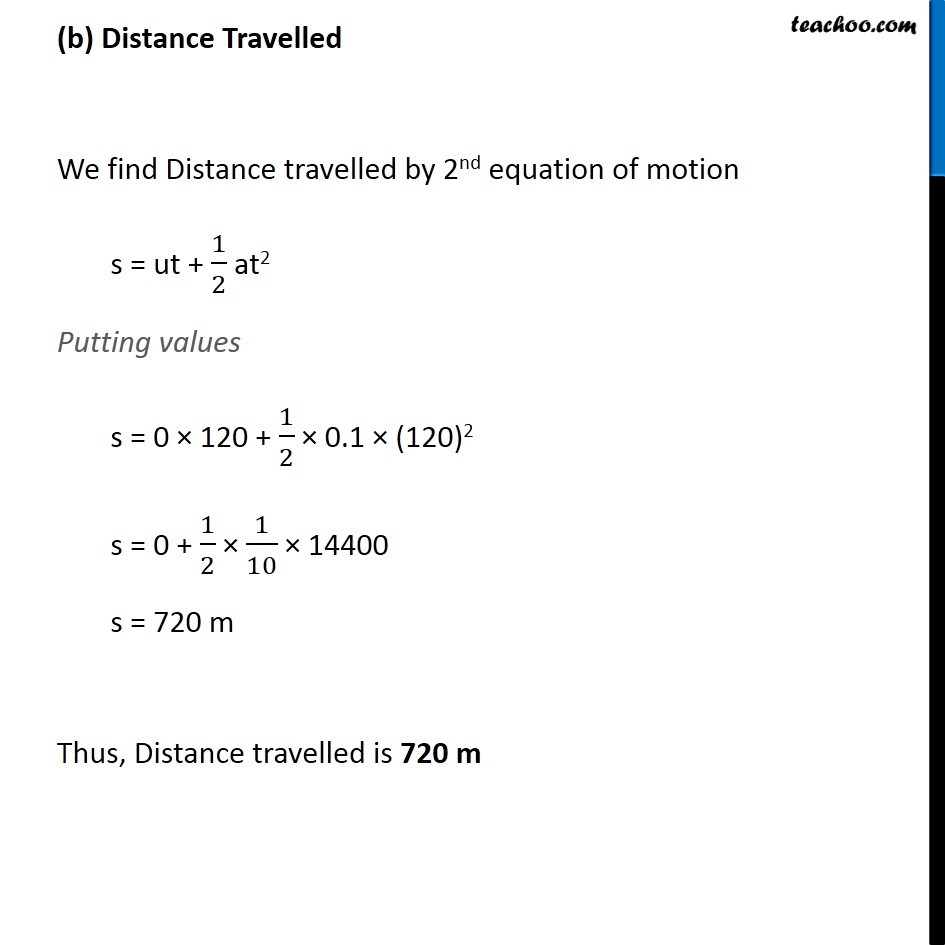Questions from Inside the chapter

Class 9
Chapter 8 Class 9 - MotionLearn in your speed, with individual attention - Teachoo Maths 1-on-1 Class

### Transcript

Page 109 Q1 A bus starting from rest moves with a uniform acceleration of 0.1 m s-2 for 2 minutes. Find (a) the speed acquired, (b) the distance travelled. Since bus starts from rest, Initial velocity = u = 0 m/s Acceleration = a = 0.1 m/s2 Time = t = 2 minutes = 2 × 60 seconds = 120 s Speed Acquired Speed acquired will be the final velocity Speed acquired = Final velocity = v Since we know u, a and t We use first equation of motion, v = u + at = 0 + 0.1 × 120 = 12 m/s Thus, Speed acquired is 12 m/s (b) Distance Travelled We find Distance travelled by 2nd equation of motion s = ut + 1/2 at2 Putting values s = 0 × 120 + 1/2 × 0.1 × (120)2 s = 0 + 1/2 × 1/10 × 14400 s = 720 m Thus, Distance travelled is 720 m